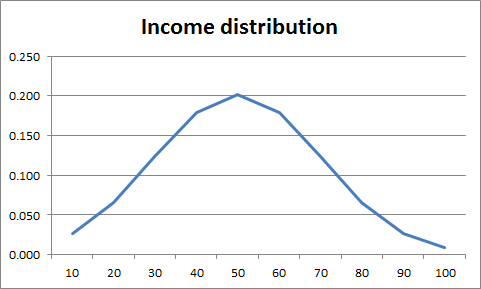14
Jan 17

## Chebyshev inequality - enigma or simplicity itself?

Let's go back to the very basics. The true probability distribution is usually unknown. This is why using separate values and probabilities is prohibited and we work with various averages. However, as you will see below, the Chebyshev inequality answers a question about behavior of certain probabilities.

### Motivation

Table 1. Income distribution

IncomePercentageP(Income>=c)Chebyshev boundBound/true
100.02715.055.05
200.0660.9732.5252.595
300.1230.9071.6831.856
400.1790.7841.2631.611
500.2020.6061.011.667
600.1790.4030.8422.089
700.1230.2250.7213.204
800.0660.1020.6316.186
900.0270.0360.56115.583
1000.0090.0090.50556.111
In Table 1, in the first two columns we have information about income distribution (income values and their probabilities or percentages of the population) in a hypothetical country H. For example, 0.027 is the proportion of the population that has income of 10 H-dollars.Figure 1. Income distribution

I simulated this distribution using the normal distribution function of Excel in such a way as to get relatively low percentages of poor and rich people, see Figure 1.

Suppose the government wants to increase the income tax on wealthy people and use the resulting tax revenue to support the low income population. The question is what part of the population will be impacted. The percentage of the population with income higher than or equal to a given cut-off level$c$ is given by

(1)$P(Income\ge c).$

For example, the government may decide to impose a higher tax on wealthy people with$Income\ge 90$, in which case the proportion of affected people will be 0.027+0.009=0.036. If the tax revenue is to be used only to support the poorest people with income of 10 H-dollars, the proportion of people who benefit from this decision can also be expressed using probability (1) because$P(Income=10)=1-P(Income\ge 20)$. It's easy to see that probability (1) is a cumulative probability: to find it, we sum all probabilities, starting from the last row up to the row in which$Income=c$. Denoting$I_j$ income levels and$p_j$ the corresponding probabilities, we have

(2)$P(Income\ge c)=\sum_{I_j\ge c}p_j.$

The third column of Table 1 contains these probabilities for all cut-off values.

Question. The true probabilities are usually unknown but the mean is normally available (to obtain the GDP per capita, just divide the GDP by the head count). In our case the mean income is 50.5. What can be said about (1) if the the cut-off value and the mean are known?

Chebyshev noticed that for those$j$ over which we sum in (2) we have$c\le I_j$ or, equivalently,$1\le I_j/c$. His answer is obtained in two steps:$P(Income\ge c)=\sum_{I_j\ge c}p_j\times 1$ (replacing 1 by$I_j/c$ can only increase the right-hand side)$\le\sum_{I_j\ge c}p_jI_j/c$ (increase the sum further by including all$j$)$\le\sum_jp_jI_j/c=\frac{1}{c}EIncome.$

Thus, we cannot find the exact value of (1) but we can give an upper bound$P(Income\ge c)\le\frac{1}{c}EIncome$. The fourth column of Table 1 contains Chebyshev bounds. The fifth column, which contains the ratios of the bounds to the true values from column 3, shows that the bounds are reasonably good for middle incomes and badly miss the mark for low and high incomes.

The above proof applies to any nonnegative random variable$X$ and positive$c$ and we state the result as the simplest form of the Chebyshev inequality:

(3)$P(X\ge c)\le\frac{1}{c}EX$.

### Extensions

1. If$X$ changes sign, its absolute value is nonetheless nonnegative, so$P(|X|\ge c)\le\frac{1}{c}E|X|$.
2. It is more interesting to bound the probability of deviation of$X$ from its mean$EX$. For this, just plug$|X-EX|$ in (3):$P(|X-EX|\ge c)\le\frac{1}{c}E|X-EX|$.
3. One more step allows us to obtain$Var(X)$ instead of$E|X-EX|$ at the right. Note that the events$|X-EX|\ge c$ and$|X-EX|^2\ge c^2$ are equivalent. Therefore$P(|X-EX|\ge c)=P(|X-EX|^2\ge c^2)\le\frac{1}{c^2}E|X-EX|^2=\frac{1}{c^2}Var(X).$

The result we have obtained$P(|X-EX|\ge c)\le \frac{1}{c^2}Var(X)$ will be referred to as the Chebyshev inequality.

### Digression

A long time ago I read a joke about P.L. Chebyshev. He traveled to Paris to give a talk named "On the optimal fabric cutout". The best Paris fashion designers gathered to listen to his presentation. They left the room after he said: For simplicity, let us imagine that the human body is ball-shaped.